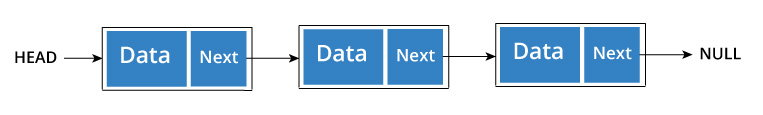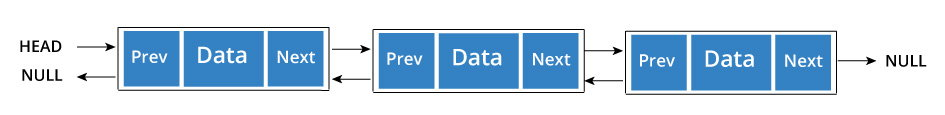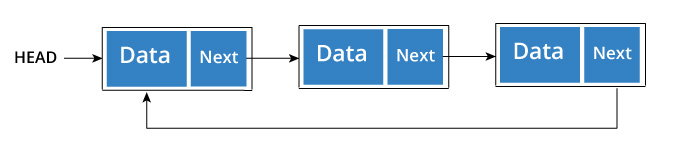Types of Linked List - Singly linked, doubly linked and circular

There are three common types of Linked List.

It is the most common. Each node has data and a pointer to the next node.Node is represented as:

struct node {
int data;
struct node *next;
}

A three member singly linked list can be created as:

/* Initialize nodes */
struct node *one = NULL;
struct node *two = NULL;
struct node *three = NULL;

/* Allocate memory */
one = malloc(sizeof(struct node));
two = malloc(sizeof(struct node));
three = malloc(sizeof(struct node));

/* Assign data values */
one->data = 1;
two->data = 2;
three->data = 3;

/* Connect nodes */
one->next = two;
two->next = three;
three->next = NULL;

/* Save address of first node in head */

We add a pointer to the previous node in a doubly linked list. Thus, we can go in either direction: forward or backward.A node is represented as

struct node {
int data;
struct node *next;
struct node *prev;
}

A three member doubly linked list can be created as

/* Initialize nodes */
struct node *one = NULL;
struct node *two = NULL;
struct node *three = NULL;

/* Allocate memory */
one = malloc(sizeof(struct node));
two = malloc(sizeof(struct node));
three = malloc(sizeof(struct node));

/* Assign data values */
one->data = 1;
two->data = 2;
three->data = 3;

/* Connect nodes */
one->next = two;
one->prev = NULL;

two->next = three;
two->prev = one;

three->next = NULL;
three->prev = two;

/* Save address of first node in head */

A circular linked list is a variation of linked list in which the last element is linked to the first element. This forms a circular loop.A circular linked list can be either singly linked or doubly linked.

• for singly linked list, next pointer of last item points to the first item
• In doubly linked list, prev pointer of first item points to last item as well.

A three member circular singly linked list can be created as:

/* Initialize nodes */
struct node *one = NULL;
struct node *two = NULL;
struct node *three = NULL;

/* Allocate memory */
one = malloc(sizeof(struct node));
two = malloc(sizeof(struct node));
three = malloc(sizeof(struct node));

/* Assign data values */
one->data = 1;
two->data = 2;
three->data = 3;

/* Connect nodes */
one->next = two;
two->next = three;
three->next = one;

/* Save address of first node in head */